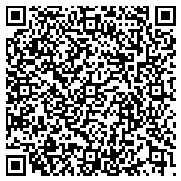#an Entity references as follows:

In geometry, the polar circle of a triangle is the circle whose center is the triangle's orthocenter and whose squared radius is where A, B, C denote both the triangle's vertices and the angle measures at those vertices, H is the orthocenter (the intersection of the triangle's altitudes), D, E, F are the feet of the altitudes from vertices A, B, C respectively, R is the triangle's circumradius (the radius of its circumscribed circle), and a, b, c are the lengths of the triangle's sides opposite vertices A, B, C respectively.Graph IRICount
http://dbpedia.org42 triples
Faceted Search & Find service v1.17_git51

Alternative Linked Data Documents: PivotViewer | ODE     Raw Data in: CXML | CSV | RDF ( N-Triples N3/Turtle JSON XML ) | OData ( Atom JSON ) | Microdata ( JSON HTML) | JSON-LD    About